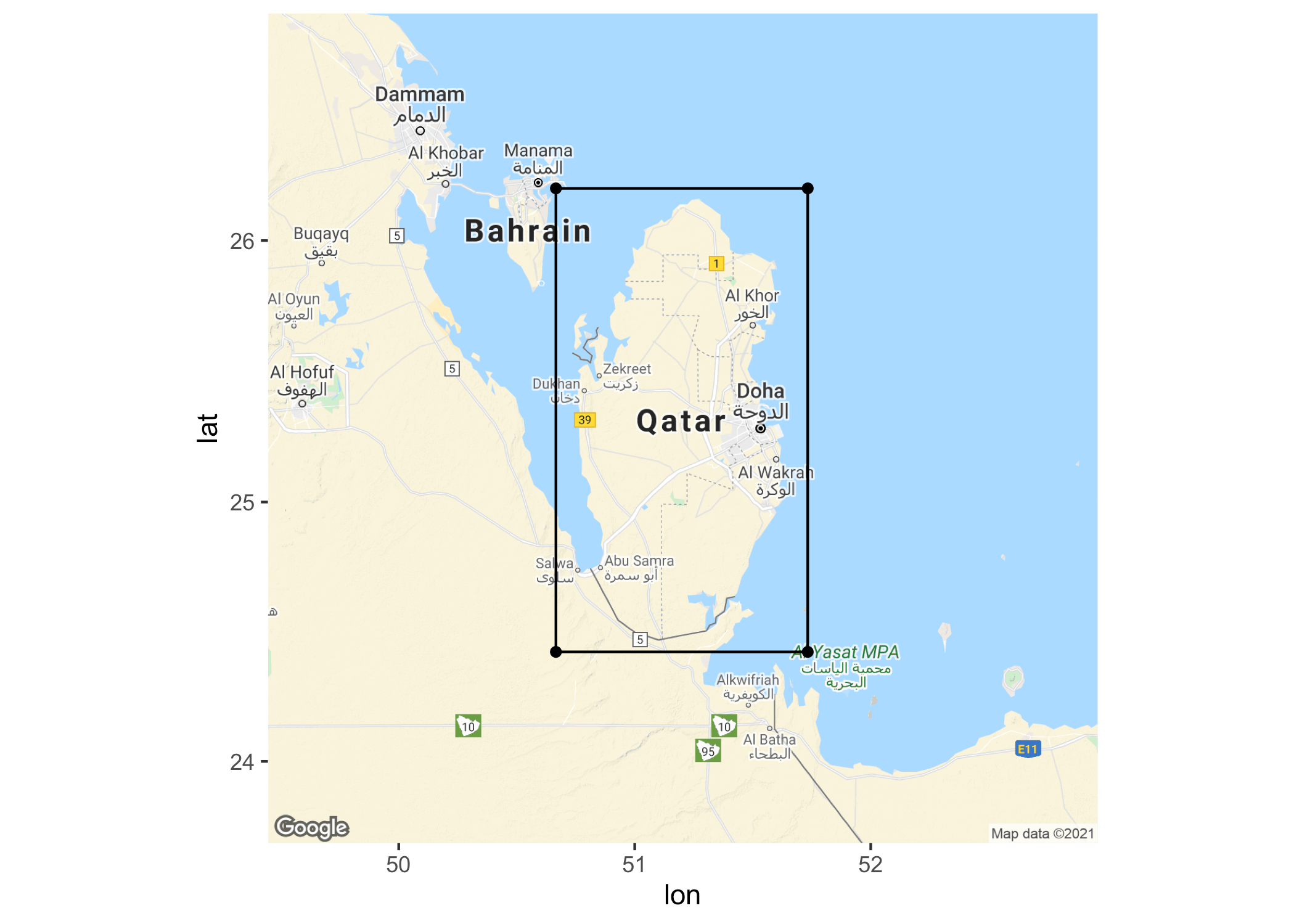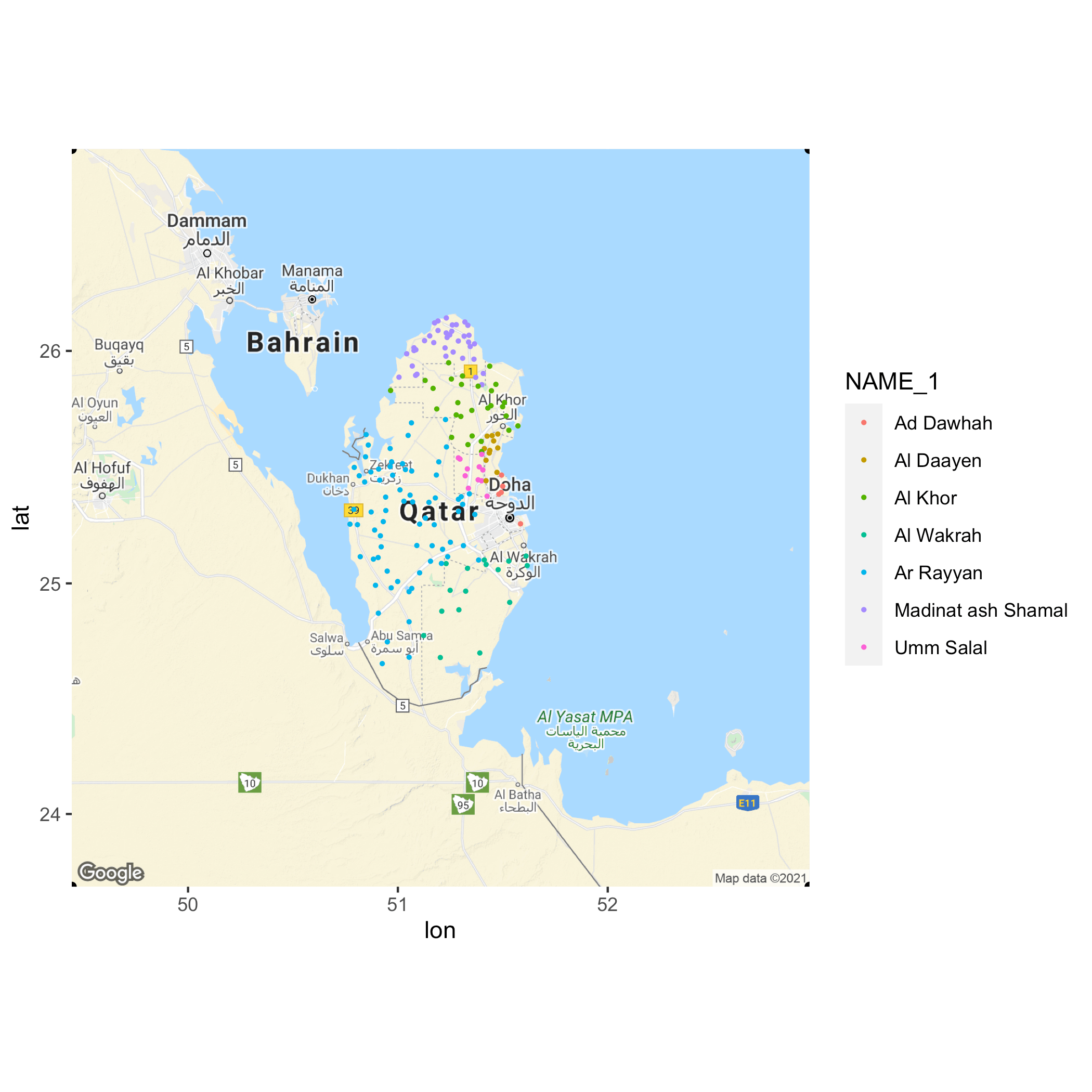Want to share your content on R-bloggers? click here if you have a blog, or here if you don't.

In this blog post, we will be exploring how I answered a question that got me my first job as a data analyst. It was an open-ended question and was supposed to be answered in a casual/theoretical way. The question was “How would you collect all cities in Qatar and how I would make sure in which municipality the cities are?”.

I took a practical approach toward the questions and made use of the Google geocoding API and coded my approach in R.

## My Approach

At first, I thought about web scraping some tables on Wikipedia and reporting the cities that way. However, I found that the tables mentioned that they were incomplete.

After that, I thought about using the Google Maps API to randomly insert latitude and longitude points into the map of Qatar. If I would randomly insert a lot of points, then eventually every city would be overlaid with a point. I then was able to use reverse geocoding with the longitude and latitude points to get information from Google about what city the point hit.

## Drawing a Square over Qatar

Firstly, I had to find out the latitude and longitude values from the edges of the square that would overlay Qatar. I used Google Maps and copy-pasted the values when right-clicking on the map.

```df_square <- dplyr::tibble(
upper_left = c(26.198624161777108, 50.66603353027927),
upper_right = c(26.198624161777108, 51.73312086696592),
lower_left = c(24.423192130919706, 50.66603353027927),
lower_right = c(24.423192130919706, 51.73312086696592)
) %>%
tibble::rownames_to_column() %>%
tidyr::pivot_longer(-rowname) %>%
tidyr::pivot_wider(names_from = rowname, values_from = value) %>%
purrr::set_names(c("name", "lat", "lon"))

df_square

## # A tibble: 4 x 3
##   name          lat   lon
##   <chr>       <dbl> <dbl>
## 1 upper_left   26.2  50.7
## 2 upper_right  26.2  51.7
## 3 lower_left   24.4  50.7
## 4 lower_right  24.4  51.7```

After transforming the data, we can use the `ggmap()` function to plot the square that overlays Qatar.

```p <- ggmap(get_googlemap(
center = c(lon = 51.2010, lat = 25.2854),
zoom = 8, scale = 2, size = c(640, 640),
maptype = "terrain",
color = "color"
))

p +
geom_point(data = df_square, aes(x = lon, y = lat)) +
geom_line(data = df_square[1:2, ], aes(x = lon, y = lat)) +
geom_line(data = df_square[3:4, ], aes(x = lon, y = lat)) +
geom_line(data = df_square[c(1, 3), ], aes(x = lon, y = lat)) +
geom_line(data = df_square[c(2, 4), ], aes(x = lon, y = lat))```## Randomly Insert Points Into the Map

Next, we need to randomly insert points into the square. We will be using the `runif()` function to randomly insert points into the square.

```# create randomly latitude and longitude values that fall within a rectangle
# that covers the entire country of Qatar
data.frame(
lat = runif(n = 5000, min = df_square\$lat, max = df_square\$lat),
lon = runif(n = 5000, min = df_square\$lon, max = df_square\$lon)
) %>%
dplyr::distinct() %>%
dplyr::as_tibble() -> lat_long_qatar

# plotting
p + geom_point(data = lat_long_qatar, aes(x = lon, y = lat), size = 0.5, alpha = 1) +
geom_point(data = df_square, aes(x = lon, y = lat)) +
geom_line(data = df_square[1:2, ], aes(x = lon, y = lat)) +
geom_line(data = df_square[3:4, ], aes(x = lon, y = lat)) +
geom_line(data = df_square[c(1, 3), ], aes(x = lon, y = lat)) +
geom_line(data = df_square[c(2, 4), ], aes(x = lon, y = lat))```For each month, Google provides us with \$200 bucks that are used towards the use of the geocoding API. Because it is “expensive” to look up a lot of points with the API we are going to remove the dots that do not fall inside the country of Qatar.

To do this we will be using the `sf` package. It’s my favorite package in R for doing geographical things. To use the package we have downloaded two Qatar shapefiles from the https://gadm.org/download_country_v3.html website. We use the country shapefile to determine what points fall in the country and what points fall outside the country.

```qatar_counrty <- readr::read_rds("shapefile_qatar/gadm36_QAT_0_sf.rds")

df_points <- sf::st_as_sf(lat_long_qatar, coords = c("lon", "lat"), crs = 4326)

valid_points <- df_points %>%
dplyr::mutate(
intersection = as.integer(sf::st_intersects(geometry, qatar_counrty)),
area = qatar_counrty\$NAME_0[intersection]
) %>%
dplyr::filter(!is.na(area)) %>%
dplyr::mutate(lon = purrr::map_dbl(geometry, 1),
lat = purrr::map_dbl(geometry, 2)) %>%
dplyr::select(lon, lat)

p + geom_point(data = valid_points, aes(x = lon, y = lat), size = 0.5, alpha = 1) +
geom_point(data = df_square, aes(x = lon, y = lat))```If we had unlimited money and computing resources, we could have skipped this step. Next, we will be using the points to do reverse geocoding with the Google Maps API. We give the `google_reverse_geocode()` function the latitude and longitude values and we get back the city of that value.

## Final Computations

```all_addresses <- vector(mode = "list", length = nrow(valid_points))
location = c(valid_points\$lat[i], valid_points\$lon[i]),
result_type = c("street_address", "postal_code", "neighborhood", "sublocality",
location_type = "approximate",
key = key,
simplify = T
)
}

~ pluck(., 1, 1)
)

valid_points_2 <- valid_points %>%
tidyr::separate(all_addresses, into = c("city", "country"), sep = ", ")```

After getting all the addresses we can now determine with the second shapefile, which outlines the municipalities, to what municipality a city belongs to.

We again use the `sf` package to determine the intersection between a point and a polygon.

```qatar_municipalities <- readr::read_rds("shapefile_qatar/gadm36_QAT_1_sf.rds")

valid_points_3 <- valid_points_2 %>%
dplyr::mutate(
intersection = as.integer(sf::st_intersects(geometry, qatar_municipalities)),
NAME_1 = qatar_municipalities\$NAME_1[intersection],
TYPE_1 = qatar_municipalities\$TYPE_1[intersection]
) %>%
dplyr::as_tibble() %>%
dplyr::distinct(city, .keep_all = TRUE) %>%
dplyr::filter(!is.na(country))

p + geom_point(data = valid_points_3, aes(x = lon, y = lat, col = NAME_1), size = 0.5, alpha = 1) +
geom_point()```And voila! The final result. That looks pretty decent and we were able to do all that in R. Pretty amazing.

## Conclusion

– With unlimited resources, this approach is very good and we can also extract public places and other spaces from the map.
– If we were to choose enough points, we can be sure to extract every single city in Qatar, given that it is registered with Google.
– A major drawback of this solution is the cost. Imagine we had to do the same thing we Russia or Canada. I would be broke by the end of the analysis.

The post The Question That Got Me My First Data Analyst Job appeared first on .